# SAT Math Multiple Choice Question 102: Answer and Explanation

### Test Information

Question: 102

12. Students in a physics class are studying how the angle at which a projectile is launched on level ground affects the projectile's hang time and horizontal range. Hang time can be calculated using the formula t =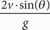,where t is the hang time in seconds, v is the initial launch velocity, θ is the projectile angle with respect to level ground, and g is the acceleration due to gravity, defined as 9.8 m/s2. Horizontal range can be calculated using the formula R =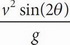, where R is the distance the projectile travels from the launch site, in feet. Which of the following gives the value of v, in terms of R, t, and θ ?

• A. v =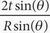• B. v =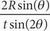• C. v =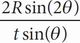• D. v =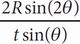t/R =/Then v =